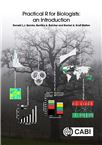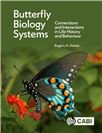Like most websites we use cookies. This is to ensure that we give you the best experience possible.

## CAB eBooks

Ebooks on agriculture and the applied life sciences from CAB International

Results per page:

## Search results

CABI Book Chapter Info### How to use this book.

This book takes a simple step-by-step approach to give a good grounding in the use of R for undergraduate/beginning postgraduate biology students. R is a freely available, open-source statistical programming environment which provides powerful statistical analysis tools and graphics outputs. This...

Author(s)
Quicke, D.; Butcher, B. A.; Welton, R. K.
ISBN
2021 CAB International (H ISBN 9781789245349)
Type
Book chapter
CABI Book Chapter Info### Statistics: choosing the right test.

Whilst this is not a book on statistics per se, one of the things R is most used for is doing statistical analyses. There are many sorts of statistical analyses and choosing the most appropriate ones can be very confusing for students. This chapter outlines the basic sorts of tests and analyses.

Author(s)
Quicke, D. L. J.; Butcher, B. A.; Welton, R. A. K.
ISBN
2021 CAB International (H ISBN 9781789245349)
Type
Book chapter
CABI Book Chapter Info### Commonly used measures and statistical tests.

There are a number of statistical tests that are frequently used, even by non-specialists. This chapter will cover tests such as Chi-squared, Fisher's exact test, Mann-Whitney U and several variations of the Student's t-test, amongst others.

Author(s)
Quicke, D. L. J.; Butcher, B. A.; Welton, R. A. K.
ISBN
2021 CAB International (H ISBN 9781789245349)
Type
Book chapter
CABI Book Chapter Info### Principal components analysis.

This chapter focuses on how to conduct a principal components analysis. To conduct principal components analysis, R has two similar built-in functions prcomp and princomp in the default stats package. Other implementations can be found in various downloadable packages, e.g. the function PCA from...

Author(s)
Quicke, D.; Butcher, B. A.; Welton, R. K.
ISBN
2021 CAB International (H ISBN 9781789245349)
Type
Book chapter
CABI Book Chapter Info### The space frame for butterfly biology systems.

This chapter focuses on the importance of the space frame in butterfly biology systems. The key roles of statistical analyses based on characteristics of samples of objects in organism spatial studies are discussed. The influence of the habitat, i.e. geology, soils, landforms, other biota and...

Author(s)
Dennis, R. L. H.
ISBN
2020 CABI (H ISBN 9781789243574)
Type
Book chapter
CABI Book Chapter Info### Ecological methods and statistics.

This chapter contains questions about the methods used by ecologists to sample and measure the biological components of ecosystems, and the statistical analysis of ecological data.

Author(s)
Rees, P. A.
ISBN
2020 CABI (H ISBN 9781789247572)
Type
Book chapter
CABI Book Info### Practical R for biologists: an introduction.

R is an open-source statistical environment modelled after the previously widely used commercial programs S and S-Plus, but in addition to powerful statistical analysis tools, it also provides powerful graphics outputs. In addition to its statistical and graphical capabilities, R is a programming...

Author(s)
Quicke, D. L. J.; Butcher, B. A.; Welton, R. A. K.
ISBN
2021 CAB International (H ISBN 9781789245349)
Type
Book
CABI Book Chapter Info### Very basic R syntax.

R is a programming language that has a huge range of inbuilt statistical and graphical functions. Firstly, this chapter shows how R works by talking you through a number of exercises, often producing graphical output, so you will get to know how to write simple code and become familiar with some of ...

Author(s)
Quicke, D. L. J.; Butcher, B. A.; Welton, R. A. K.
ISBN
2021 CAB International (H ISBN 9781789245349)
Type
Book chapter
CABI Book Chapter Info### Plotting biological data in various ways.

This chapter introduces plotting line graphs, bar charts, pie charts, box and whisker plots. It will troubleshoot the main areas where you are likely to encounter problems. It will show how to create log plots, add legends, error bars, notches and confidence limits, and introduce confidence limits...

Author(s)
Quicke, D. L. J.; Butcher, B. A.; Welton, R. A. K.
ISBN
2021 CAB International (H ISBN 9781789245349)
Type
Book chapter
CABI Book Chapter Info### Analysis of variance (ANOVA).

Analysis of variance is used to analyze the differences between group means in a sample, when the response variable is numeric (real numbers) and the explanatory variable(s) are all categorical. Each explanatory variable may have two or more factor levels, but if there is only one explanatory...

Author(s)
Quicke, D.; Butcher, B. A.; Welton, R. K.
ISBN
2021 CAB International (H ISBN 9781789245349)
Type
Book chapter

More results…

More results…

More results…